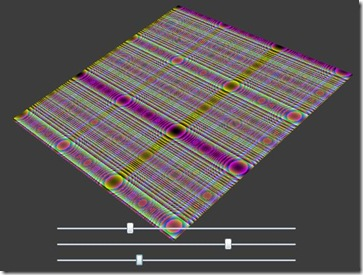# Silverlight 3 Beta 新特性解析（2）-Graphics篇

## 本篇主要内容：

• 如何利用新的Bitmap API来创建我们自己的图像
• 透视3D图像(Perspective 3D Graphic)
• 像素模糊和投影效果
• Element-To-Element Binding

## Bitmap API的写图像功能：

`   1: public sealed class WriteableBitmap : BitmapSource`
`   2: {`
`   3:     public WriteableBitmap(BitmapSource source);`
`   4:     public WriteableBitmap(int pixelWidth, int pixelHeight, PixelFormat format);`
`   5:     public int this[int index] { get; set; }`
`   6:     public void Invalidate();`
`   7:     public void Lock();`
`   8:     public void Render(UIElement element, Transform transform);`
`   9:     public void Unlock();`
`  10: }`

1. 实例化WriteableBitmap
2. 调用Lock方法
3. 写像素点
4. 调用Invalidate方法
5. 最后是调用Unlock方式来释放所有的Buffer并准备显示

`   1: private WriteableBitmap BuildTheImage(int width, int height)`
`   2: {`
`   3:     WriteableBitmap wBitmap=new WriteableBitmap(width,height,PixelFormats.Bgr32);`
`   4:     wBitmap.Lock();`
`   5:  `
`   6:     for (int x = 0; x < width; x++)`
`   7:     {`
`   8:         for (int y = 0; y < height; y++)`
`   9:         {`
`  10:             byte[] brg = new byte;`
`  11:             brg=(byte)(Math.Pow(x,2) % 255);   //Blue, B`
`  12:             brg = (byte)(Math.Pow(y,2) % 255); //Green, G`
`  13:             brg = (byte)((Math.Pow(x, 2) + Math.Pow(y, 2)) % 255); //Red, R`
`  14:             brg = 0;`
`  15:  `
`  16:             int pixel = BitConverter.ToInt32(brg, 0);`
`  17:  `
`  18:             wBitmap[y * height + x] = pixel;`
`  19:         }`
`  20:     }`
`  21:  `
`  22:     wBitmap.Invalidate();`
`  23:     wBitmap.Unlock();`
`  24:  `
`  25:     return wBitmap;`
`  26: }`

画出来的图像如下图所示

## 透视3D效果

`   1: "Img">`
`   2:     `
`   3:         "ImageProjection" RotationX="-20"/>`
`   4:     `
`   5: `

## 像素模糊和投影效果

`   1: `
`   2:     `
`   3:         "20"/>`
`   4:     `
`   5: `

Color属性用来设置投影的颜色，默认颜色是黑色

Direction属性用来控制投影方向，值为0时代表投影到控件的正右方，以逆时针的形式来增大投影角度

默认值为315，值的范围只能在0~360之间

Opacity属性用来控制边缘的透明度，其使用而控件的Opacity属性一样

`   1: `
`   2:     `
`   3:         "5" Color="White" Opacity="0.7" ShadowDepth="7"/>`
`   4:     `
`   5: `

## Element-To-Element Binding：

`   1: "400" Height="400" x:Name="Img">`
`   2:     `
`   3:         "ImageProjection"/>`
`   4:     `
`   5: `
`   6: "PlotX" Minimum="-90" Maximum="90" Value="{Binding RotationX, Mode=TwoWay, ElementName=ImageProjection}"/>`
`   7: "PlotY" Minimum="-90" Maximum="90" Value="{Binding RotationY, Mode=TwoWay, ElementName=ImageProjection}"/>`
`   8: "PlotZ" Minimum="-90" Maximum="90" Value="{Binding RotationZ, Mode=TwoWay, ElementName=ImageProjection}"/>`0
0